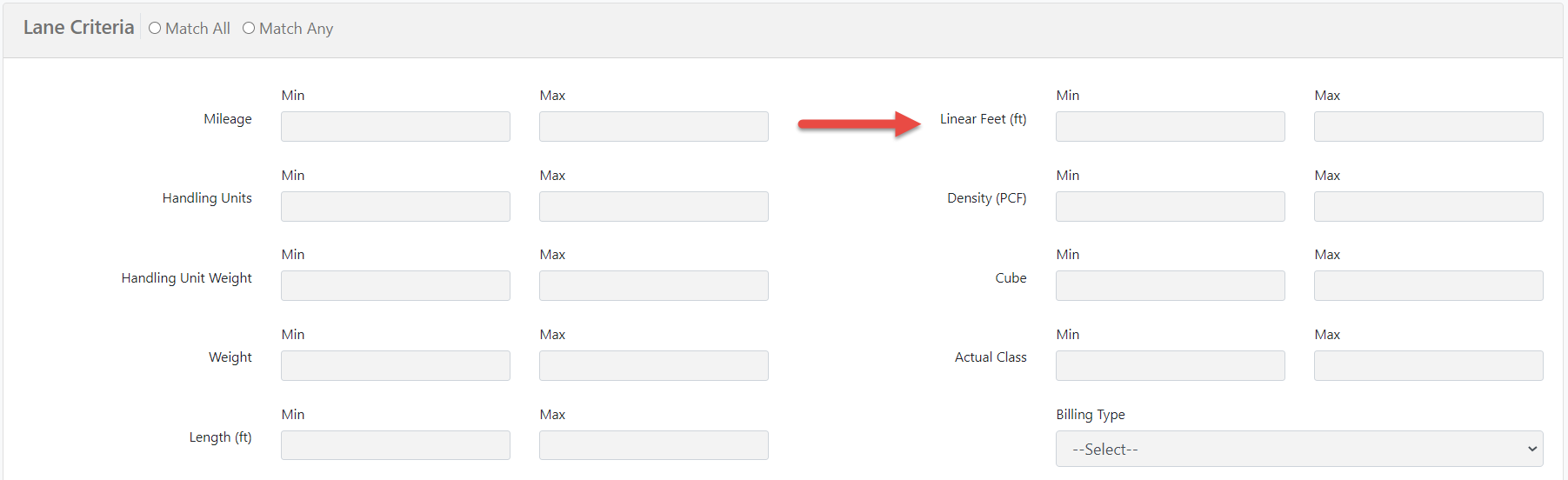Knowledge Base

# Linear Feet Calculator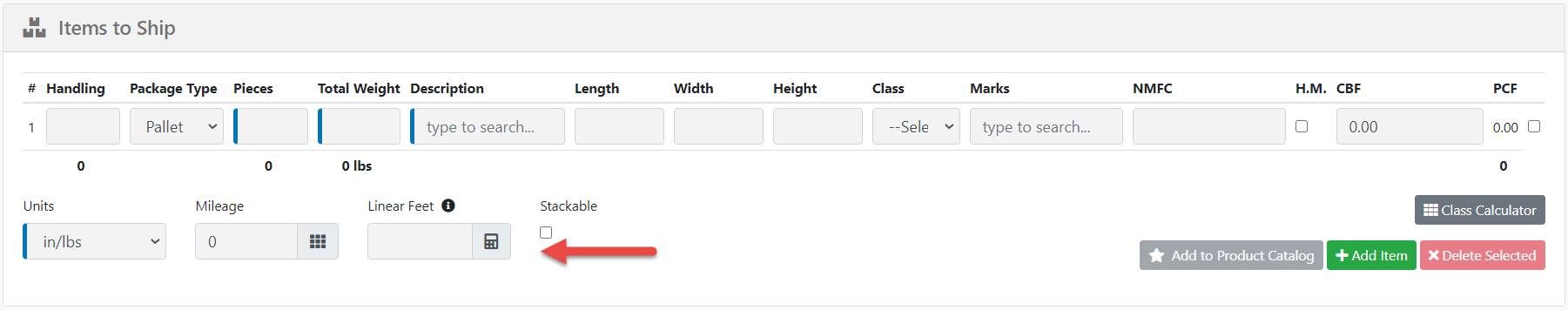The built-in Linear Feet calculator can estimate the Linear Footprint of a shipment using the total handling units, length, width, height, and stackability. The calculator will arrange the freight using the Length x Width orientation provided by the user and then measure the total linear feet with the following criteria:

• Maximum Truck length of 48'
• Maximum Truck width of 96"
• Maximum Truck height of 96"
• Allows for stacking items 2 rows high
• Up to 75 handling units
• Rounds Linear Feet to the next whole number (i.e. 11.5 ft is the same as 12 ft)

A visual representation of this for example would be: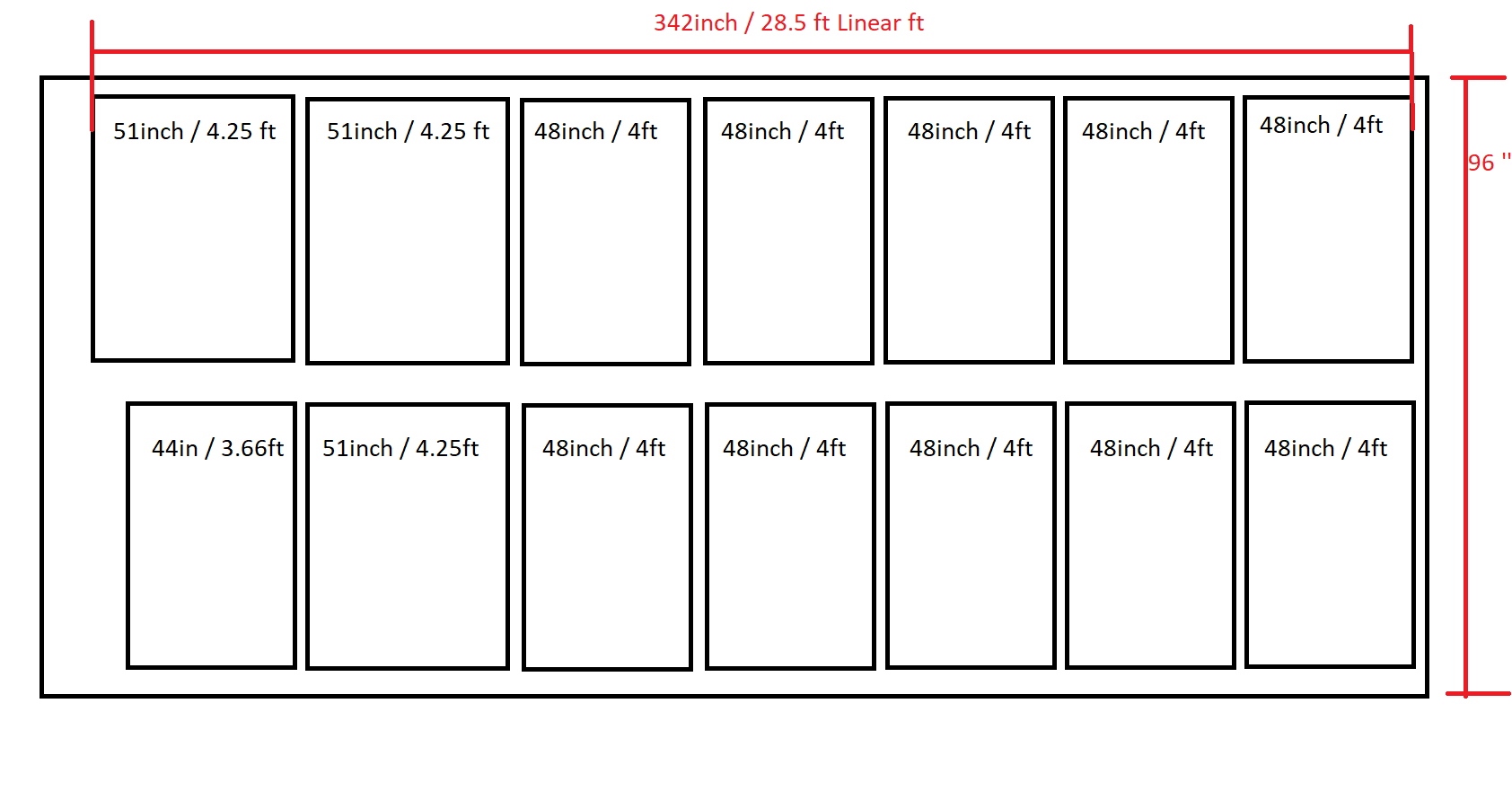This calculation will happen automatically when the user provides the number of handling units, length, width, and height. The stackable attribute is optional and will only count if selected.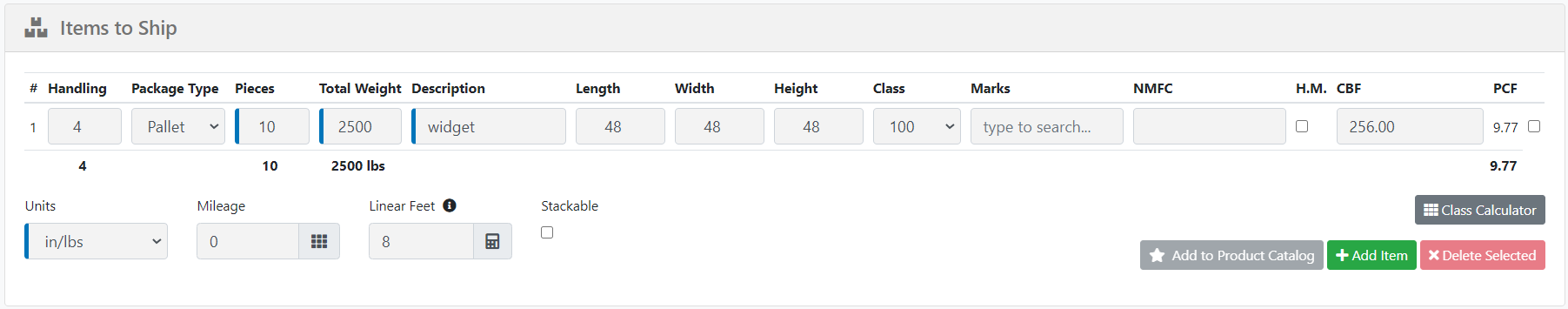If one of the handling unit, length, width, or height parameters is missing, the system will show a yellow info icon indicating which value is missing to complete the calculation.You can manually override the estimated linear feet calculation by adding your own value into the linear feet field. Once you've added your own the calculator will turn yellow and the system will no longer update on it's own. If you click the calculator the system will then re-calculate and override your manual selection.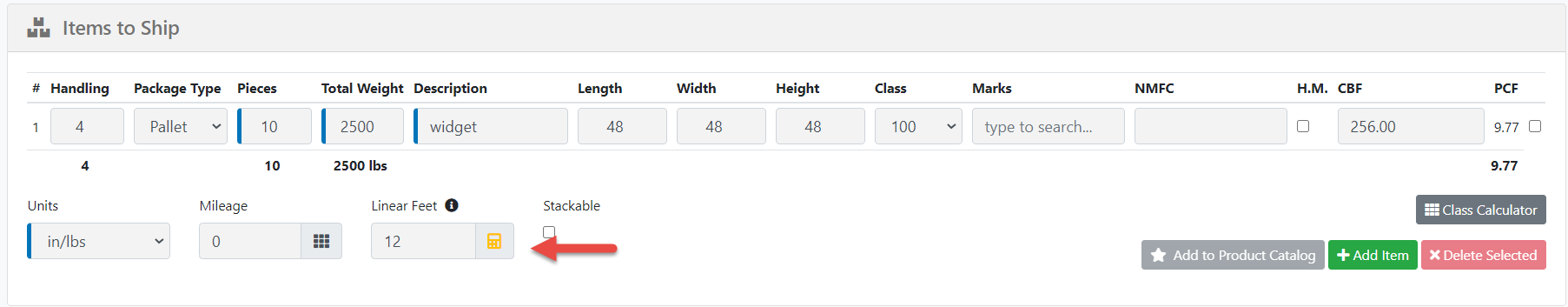Lane Criteria

If you have any added lane criteria we will now use the linear feet calculation to determine the rate. If a linear feet calculation is not provided we will attempt to fall back on the commodity details and calculate the linear feet in the background. If the linear feet and dimensions are both empty the rate simply will not meet the lane criteria provided.